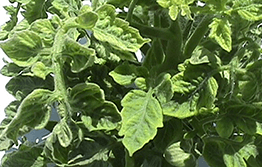```int(43)
bool(false)
int(0)
```
```int(1306)
bool(false)
int(43)
```
```int(2309)
bool(false)
int(43)
```
```int(55)
bool(false)
int(43)
```
```int(1307)
bool(false)
int(43)
```
```int(41)
bool(false)
int(0)
```
```int(60)
bool(false)
int(41)
```
```int(1311)
bool(false)
int(41)
```
```int(1371)
bool(false)
int(41)
```
```int(2576)
bool(false)
int(41)
```
```int(406)
bool(false)
int(41)
```
```int(42)
bool(false)
int(0)
```
```int(1304)
bool(false)
int(42)
```
```int(1303)
bool(false)
int(42)
```
```int(407)
bool(false)
int(42)
```
```int(62)
bool(false)
int(42)
```
```int(51)
bool(false)
int(42)
```
```int(2587)
bool(false)
int(42)
```
```int(3161)
bool(false)
int(42)
```
```int(3163)
bool(false)
int(42)
```
```int(3164)
bool(false)
int(42)
```
```int(3165)
bool(false)
int(42)
```
```int(3166)
bool(false)
int(42)
```
```int(3167)
bool(false)
int(42)
```
```int(3168)
bool(false)
int(42)
```
```int(3169)
bool(false)
int(42)
```
```int(3170)
bool(false)
int(42)
```
```int(3171)
bool(false)
int(42)
```
```int(3172)
bool(false)
int(42)
```
```int(3173)
bool(false)
int(42)
```
```int(3174)
bool(false)
int(42)
```
```int(2951)
bool(false)
int(0)
```
```int(2952)
bool(false)
int(2951)
```
```int(2953)
bool(false)
int(2951)
```
```int(2954)
bool(false)
int(2951)
```
```int(2955)
bool(false)
int(2951)
```Tomato yellow leaf curl virus (TYLCV) causes a devastating disease complex of tomato in tropical and warm temperate regions, resulting in crop losses of up to 100 per cent.

The main host of TYLCV is tomato. A range of other hosts includes capsicum, eggplant, lisianthus, poinsettia, beans, and black nightshade.

Symptoms of TYLCV vary depending on the growth stage at the time of initial infection, environmental conditions and cultivars.

In tomatoes, symptoms include severe stunting, marked reduction in leaf size, upward cupping, chlorosis of leaf margins, mottling, flower abscission and significant yield reduction.

Symptoms in common bean include leaf thickening, leaf crumpling, upward curling of leaves, abnormal lateral shoot proliferation, deformation and reduction in the number of pods.

TYLCV is found in Queensland and Northern Territory. If you think you have seen plants with signs of TYLCV anywhere else in Australia, call 1800 084 881.TYLCV can be spread on infected plants or by the insect silverleaf whitefly Bemisia tabaci biotype B.